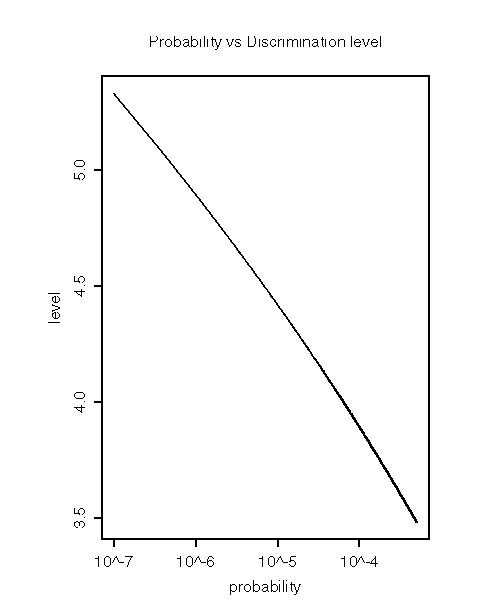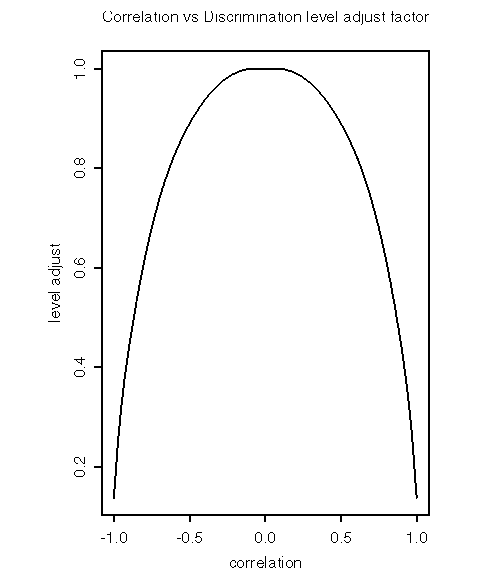Despiking algorithm

## Description of our Despiking Algorithm

The ISFF despiking algorithm is taken from the paper, "A Statistical Data Screening Procedure," by Jorgen Hojstrup, Meas. Sci. Technol., 4, 1993, pg 153-157.

A data point is detected as a spike if it deviates from a forecasted point by a discrimination level, L, times the standard deviation: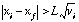Where xi is the ith data point, and xf = xi-1 * ci + ( 1 - ci ) * mi, is the forecasted data point.

For computing efficiency, the mean, mi, auto correlation, ci, and variance, vi, are approximated by running statistics: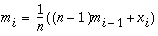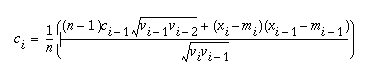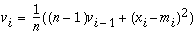The statistics memory size, n, is adjusted periodically: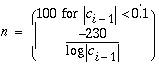The input parameters to the despiker are the minimum probability of a spike, usually 1e-5, and a level adjustment factor, usually 2.5. Choosing a minimum probability of a spike selects an initial discrimination level, based on a Gaussian distribution. See plot of Discrimination Level vs Chosen Probability. Due to the finite size of the statistical sample, the discrimination level is then multiplied by the adjustment factor. The discrimation level is then periodically updated (every 25 points) by a factor based on the correlation, see plot of Correlation vs Discrimination level adjust factor.

L = [initial level] * [level adjustment] * [correlation factor]